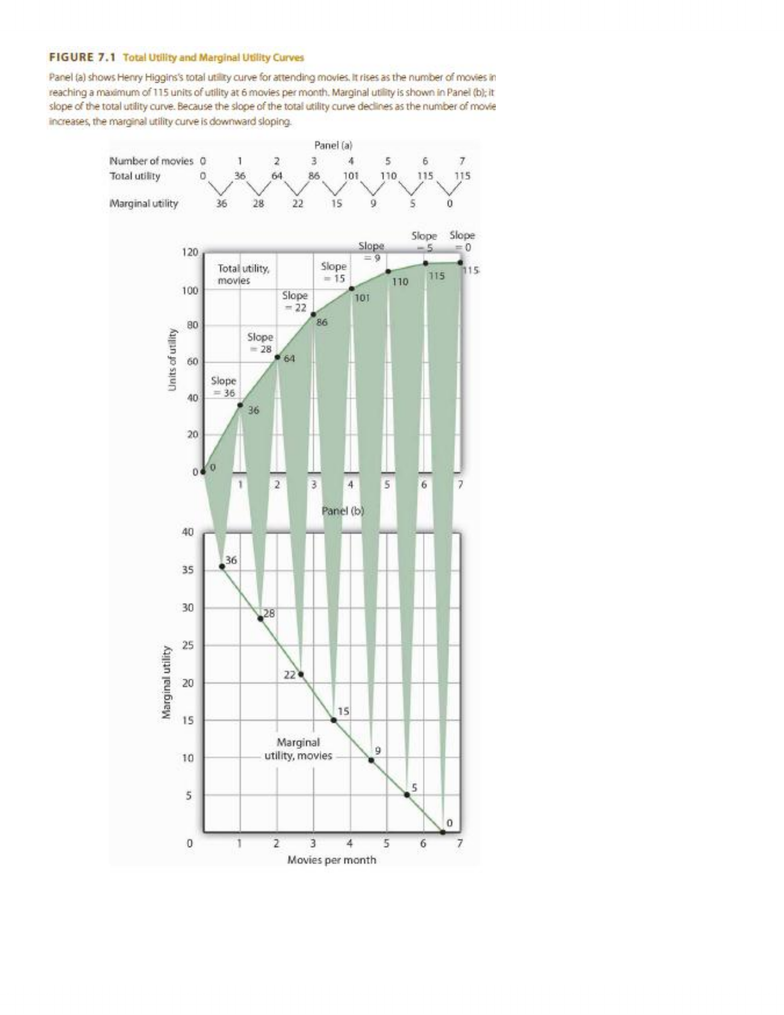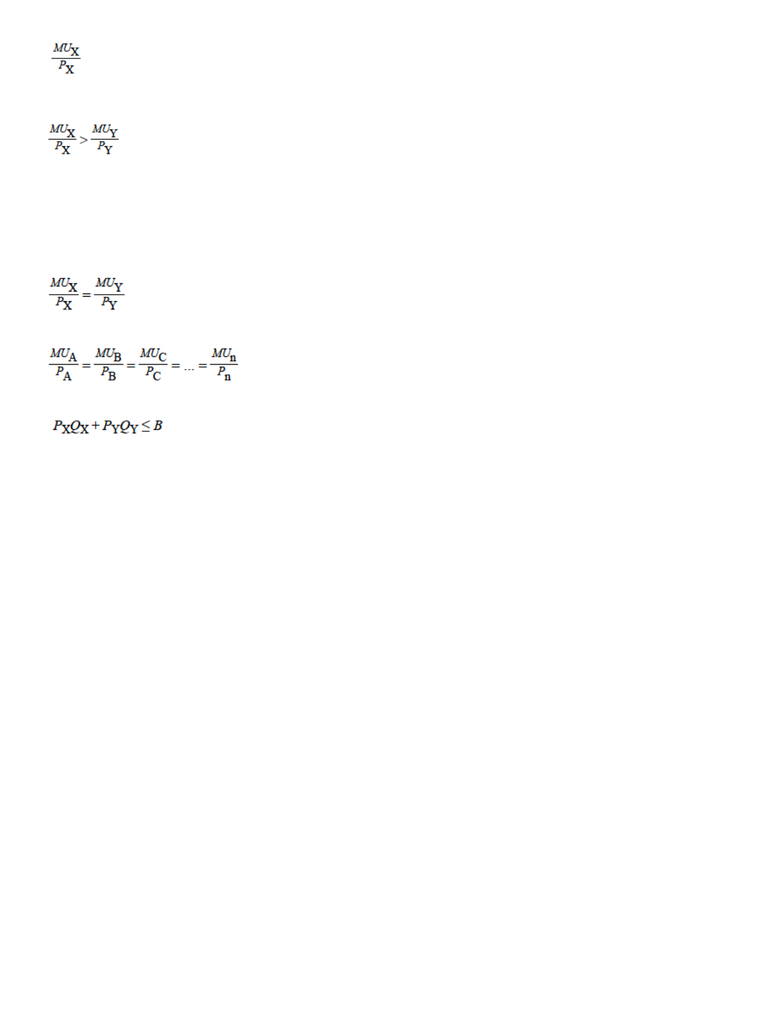Study Guides (390,000)
CA (150,000)
UW (7,000)
ECON (400)
ECON101 (100)

ECON101 Study Guide - Indifference Curve, Budget Constraint, Marginal Cost

Department
Economics
Course Code
ECON101
Professor
Lutz- Alexander Busch

This preview shows pages 1-2. to view the full 7 pages of the document.Total utility curve slopes down
Marginal utility curve slopes down

Only pages 1-2 are available for preview. Some parts have been intentionally blurred.Utility maximizing condition
Utility gained = marginal cost of X / price of X
As the consumer buys more of good X and less of good Y, marginal utilities of the two goods will change.
The law of diminishing marginal utility tells us that the marginal utility of good X will fall as the consumer
consumes more of it; the marginal utility of good Y will rise as the consumer consumes less of it
When the two sides are equal, total utility will be maximized
In terms of the marginal decision rule, the consumer will have achieved a solution at which the marginal benefit of
the activity (spending more on good X) is equal to the marginal cost
Utility maximization requires that the ratio of marginal utility to price be equal
For budget constraint for two goods X and Y
Px and Py are the prices of goods X and Y
Qx and Qy are the quantities of good X and good Y
Total expenditures on goods X and Y (on the left side of the equation) cannot exceed B
You're Reading a Preview

Unlock to view full version